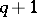# Ovoid(2)

(diff) ← Older revision | Latest revision (diff) | Newer revision → (diff)
Jump to: navigation, search

ovaloid

A setof points in some space which is intersected by an arbitrary straight line in at most two points, and such that the tangents toat each of its points cover exactly a hyperplane. In projective space a non-ruled quadric is an ovoid. This term is mainly used in finite geometries.

In finite projective spaces of dimension greater than three, ovoids do not exist. In three-dimensional spaces of order, an ovoid is a maximal-cap (cf. Cap) and consists ofpoints, and for oddany ovoid is an elliptic quadric (see ). In a plane of order, an ovoid is called an oval, and consists ofpoints. In a Desarguesian plane of odd order, any oval is uniquely representable as a non-degenerate conic over a Galois field (see ).

How to Cite This Entry:
Ovoid(2). Encyclopedia of Mathematics. URL: http://encyclopediaofmath.org/index.php?title=Ovoid(2)&oldid=17115
This article was adapted from an original article by V.V. Afanas'ev (originator), which appeared in Encyclopedia of Mathematics - ISBN 1402006098. See original article